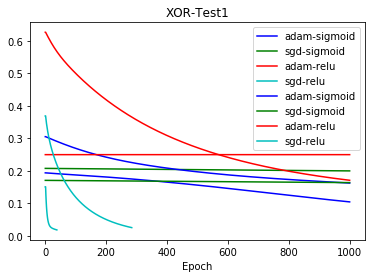# 3.20. Experiments¶

This notebook documents the use of the Experiment class for running many experiments, and comparing the results.

In :

import conx as cx

Using TensorFlow backend.
Conx, version 3.6.0


First, we create an experiment object:

In :

exp = cx.Experiment("XOR-Test1")


Experiment takes a function, and any number of variations of values.

The function takes whatever argumnets you wish, creates a network, trains it, and returns the network and a category describing the run:

In :

def function(optimizer, activation, **options):
category = "%s-%s" % (optimizer, activation)
print("category %s running..." % category)
net = cx.Network("XOR", 2, 2, 1, activation=activation)
net.compile(error="mse", optimizer=optimizer)
net.dataset.append_by_function(2, (0, 4), "binary", lambda i,v: [int(sum(v) == len(v))])
net.train(report_rate=10000, verbose=0, plot=False, **options)
return category, net


Then we run a number of trials (perhaps just 1). Notice that each argument should be given as a list. The total number of runs per trial is the product of the lengths of the arguments.

In :

exp.run(function,
trials=2,
epochs=,
accuracy=[0.8],
tolerance=[0.2],
activation=["sigmoid", "relu"],
dir="/tmp/")

category adam-sigmoid running...
category sgd-sigmoid running...
category sgd-relu running...
category sgd-sigmoid running...
category sgd-relu running...


The results is a list of (category, network-name) pairs:

In :

exp.results

Out:

[('adam-sigmoid', '/tmp/XOR-Test1-00001-00001'),
('sgd-sigmoid', '/tmp/XOR-Test1-00001-00002'),
('sgd-relu', '/tmp/XOR-Test1-00001-00004'),
('sgd-sigmoid', '/tmp/XOR-Test1-00002-00002'),
('sgd-relu', '/tmp/XOR-Test1-00002-00004')]


Often, you may wish to plot the results of learning. This may take some time, as the function will re-load each network:

In :

exp.plot("loss")Notice that each category has its own color.

There is also a generic apply method for calling a function with each of the (category, network-names). Ususally, you would probably want to re-load the network, and perform some operation in the function.

In :

exp.apply(lambda category, exp_name: (category, exp_name))

Out:

[('adam-sigmoid', '/tmp/XOR-Test1-00001-00001'),
('sgd-sigmoid', '/tmp/XOR-Test1-00001-00002'),